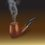# Puzzling Electromagnetism

And now, I post a question for all those people here who love the subject of electromagnetism. Well, undergraduates and post-graduates can easily answer this question, but I want school students to mull over it.

Let us consider an idealized straight conductor wire carrying current. Also let us consider a wooden lift which contains a free electron. The lift travels parallel to the current carrying wire thus creating a motion of the free electron with respect to the wire. Let us also assume that this free electron moves parallel to the electrons in the wire and with the same velocity as that of those in the wire. Clearly the conductor which carries current sets up a magnetic field (which we determine using the right-hand-thumb rule or corkscrew rule) and the electron moving in the magnetic field is expected to spiral up the wire in a helical path. But suppose you are inside the lift. What will you see? As the free electron inside the lift and those in the wire move in same direction with the same velocity, you will see that the electrons inside the conductor are at rest. That means the conductor is no longer carrying current, hence no magnetic field, and hence there is no question of spiralling up the conductor in a helical path. Instead the free electrons inside the conductor wire would then seem to repel the one inside the lift inside which you are standing. So, which one of the two observations is correct? Is the second observation correct? Think over it.Note by Kuldeep Guha Mazumder
5 years, 3 months ago

This discussion board is a place to discuss our Daily Challenges and the math and science related to those challenges. Explanations are more than just a solution — they should explain the steps and thinking strategies that you used to obtain the solution. Comments should further the discussion of math and science.

When posting on Brilliant:

• Use the emojis to react to an explanation, whether you're congratulating a job well done , or just really confused .
• Ask specific questions about the challenge or the steps in somebody's explanation. Well-posed questions can add a lot to the discussion, but posting "I don't understand!" doesn't help anyone.
• Try to contribute something new to the discussion, whether it is an extension, generalization or other idea related to the challenge.

MarkdownAppears as
*italics* or _italics_ italics
**bold** or __bold__ bold
- bulleted- list
• bulleted
• list
1. numbered2. list
1. numbered
2. list
Note: you must add a full line of space before and after lists for them to show up correctly
paragraph 1paragraph 2

paragraph 1

paragraph 2

[example link](https://brilliant.org)example link
> This is a quote
This is a quote
    # I indented these lines
# 4 spaces, and now they show
# up as a code block.

print "hello world"
# I indented these lines
# 4 spaces, and now they show
# up as a code block.

print "hello world"
MathAppears as
Remember to wrap math in $$ ... $$ or $ ... $ to ensure proper formatting.
2 \times 3 $2 \times 3$
2^{34} $2^{34}$
a_{i-1} $a_{i-1}$
\frac{2}{3} $\frac{2}{3}$
\sqrt{2} $\sqrt{2}$
\sum_{i=1}^3 $\sum_{i=1}^3$
\sin \theta $\sin \theta$
\boxed{123} $\boxed{123}$

Sort by:

Relativistic effects.

- 5 years, 3 months ago

As far as I know, Einstein proved that the electromagnetic forces are relativistic invariant. That means that no matter what relativistic frame of reference you choose, you must reach the same conclusions from all of them.

For instance, taking your fisrt frame of reference, let's say the wire is in the $x$ axis of the space. Then the lift moves in the direction of $\hat i$ inside the $xy$ plane, and the magnetic field generated by the wire has a direction of $\hat k$ in the $xy$ plane. Therefor, the magnetic force over the lift has a direction of $-\hat i \times \hat k = \hat j$, that means the lift is in fact being repelled by the wire.

However a more deep analysis must be done in order to show that results are exactly the same under both frames of reference.

- 5 years, 3 months ago

Both of you are absolutely correct but I am a bit dissatisfied as both of you know the reason..or at least have heard of it..I wanted someone to comment (if not answer) on the problem, who doesn't know about relativity, etc., etc. .. But still, congratulations. The basic essence of relativistic invariance of the laws of electromagnetism is that, the cause of an observed phenomenon may differ in different frames, but the results turn out to be the same..:-)

- 5 years, 3 months ago